# NCERT Solutions for Class 11 Physics chapter-3 Motion In A Straight Line

NCERT Solutions for class-11 Physics Chapter 3 Motion In A Straight Line is prepared by our senior and renowned teachers of Physics Wallah primary focus while solving these questions of class-11 in NCERT textbook, also do read theory of this Chapter 3 Motion In A Straight Line while going before solving the NCERT questions. You can download NCERT solution of all chapters from Physics Wallah in PDF.

### Chapter 3 Motion In A Straight Line

Question 1.In which of the following examples of motion, can the body be considered approximately a point object:
a. a railway carriage moving without jerks between two stations.
b.  a monkey sitting on top of a man cycling smoothly on a circular track.
c.  a spinning cricket ball that turns sharply on hitting the ground.
d.  a tumbling beaker that has slipped off the edge of a table.

Solution :
a,b
a. The size of a carriage is very small as compared to the distance between two stations. Therefore, the carriage can be treated as a point sized object.
b. The size of a monkey is very small as compared to the size of a circular track. Therefore, the monkey can be considered as a point sized object on the track.
c. The size of a spinning cricket ball is comparable to the distance through which it turns sharply on hitting the ground. Hence, the cricket ball cannot be considered as a point object.
d. The size of a beaker is comparable to the height of the table from which it slipped. Hence, the beaker cannot be considered as a point object.

Question 2.The position-time (x-t) graphs for two children A and B returning from their school O to their homes P and Q respectively are shown in Fig. 3.19. Choose the correct entries in the brackets below;
a. (A/B) lives closer to the school than (B/A)
b. (A/B) starts from the school earlier than (B/A)
c.  (A/B) walks faster than (B/A)
d.  A and B reach home at the (same/different) time
e. (A/B) overtakes (B/A) on the road (once/twice).

Solution :
a. A lives closer to school than B.
b.  A starts from school earlier than B.
c. B walks faster than A.
d.  A and B reach home at the same time.
e. B overtakes A once on the road.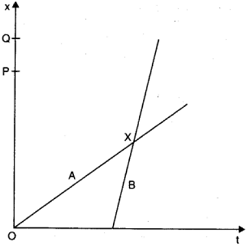Explanation:
a. In the given x–t graph, it can be observed that distance OP < OQ. Hence, the distance of school from the A’s home is less than that from B’s home.
b.  In the given graph, it can be observed that for x = 0, t = 0 for A, whereas for x = 0, t has some finite value for B. Thus, A starts his journey from school earlier than B.
c. In the given x–t graph, it can be observed that the slope of B is greater than that of A. Since the slope of the x–t graph gives the speed, a greater slope means that the speed of B is greater than the speed A.
d.  It is clear from the given graph that both A and B reach their respective homes at the same time.
e. B moves later than A and his/her speed is greater than that of A. From the graph, it is clear that B overtakes A only once on the road.

Question3.A woman starts from her home at 9.00 am, walks with a speed of 5 km h–1 on a straight road up to her office 2.5 km away, stays at the office up to 5.00 pm, and returns home by an auto with a speed of 25 km h–1. Choose suitable scales and plot the x-t graph of her motion.

Solution :
Distance covered while walking = 2.5 km.
Speed while walking = 5 km/h
Time taken to reach office while walking = (2.5/5 ) h=1/2 h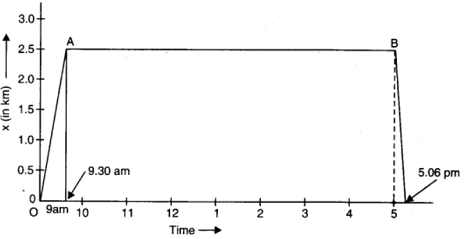If O is regarded as the origin for both time and distance, then at t = 9.00 am, x = 0
and at t = 9.30 am, x = 2.5 km
OA is the x-t graph of the motion when the woman walks from her home to office. Her stay in the office from 9.30 am to 5.00 pm is represented, by the straight line AB in the graph.
Now, time taken to return home by an auto = 2.5/5 h =1/10 h =6 minute
So, at t = 5.06 pm, x = 0
This motion is represented by the straight line BC in the graph. While drawing the x-t graph, the scales chosen are as under:
Along time-axis, one division equals 1 hour.
Along positive-axis, one division equals 0.5 km.

Question 4.A drunkard walking in a narrow lane takes 5 steps forward and 3 steps backward, followed again by 5 steps forward and 3 steps backward, and so on. Each step is 1 m long and requires 1 s. Plot the x-t graph of his motion. Determine graphically and otherwise how long the drunkard takes to fall in a pit 13 m away from the start.

Solution :
Distance covered with 1 step = 1 m
Time taken = 1 s
Time taken to move first 5 m forward = 5 s
Time taken to move 3 m backward = 3 s
Net distance covered = 5 – 3 = 2 m
Net time taken to cover 2 m = 8 s
Drunkard covers 2 m in 8 s.
Drunkard covered 4 m in 16 s.
Drunkard covered 6 m in 24 s.
Drunkard covered 8 m in 32 s.
In the next 5 s, the drunkard will cover a distance of 5 m and a total distance of 13 m and falls into the pit.
Net time taken by the drunkard to cover 13 m = 32 + 5 = 37 s
The x-t graph of the drunkard’s motion can be shown as: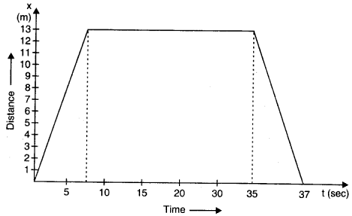Question 5.A jet airplane travelling at the speed of 500 km h–1 ejects its products of combustion at the speed of 1500 km h–1 relative to the jet plane. What is the speed of the latter with respect to an observer on ground?

Solution :
Speed of the jet airplane, vjet = 500 km/h
Relative speed of its products of combustion with respect to the plane,
vsmoke = – 1500 km/h
Speed of its products of combustion with respect to the ground = v′smoke
Relative speed of its products of combustion with respect to the airplane,
vsmoke = v′smoke – vjet
– 1500 = v′smoke – 500
v′smoke = – 1000 km/h
The negative sign indicates that the direction of its products of combustion is opposite to the direction of motion of the jet airplane.

Question 6.A car moving along a straight highway with a speed of 126 km h–1 is brought to a stop within a distance of 200 m. What is the retardation of the car (assumed uniform), and how long does it take for the car to stop?

Solution :

The initial velocity of the car = u

Final velocity of the car = v

Distance covered by the car before coming to rest = 200 m

Using the equation,

v = u + at

t = (v – u)/a = 11.44 sec.

Therefore, it takes 11.44 sec for the car to stop.

Question 7.Two trains A and B of length 400 m each are moving on two parallel tracks with a uniform speed of 72 km h–1 in the same direction, with A ahead of B. The driver of B decides to overtake A and accelerates by 1 m/s2. If after 50 s, the guard of B just brushes past the driver of A, what was the original distance between them?

Solution :

Length of the train A and B = 400 m

Speed of both the trains = 72 km/h = 72 x (5/18) = 20m/s

Using the relation, s = ut + (1/2)at2

Distance covered by the train B

SB = uBt + (1/2)at2

Acceleration, a = 1 m/s

Time = 50 s

SB = (20 x 50) + (1/2) x 1 x (50)2

= 2250 m

Distance covered by the train A

SA = uAt + (1/2)at2

Acceleration, a = 0

SA = uAt  = 20 x 50 = 1000 m

Therefore, the original distance between the two trains = SB – SA = 2250 – 1000 = 1250 m

Question 8.On a two-lane road, car A is travelling with a speed of 36 km h–1. Two cars B and C approach car A in opposite directions with a speed of 54 km h–1 each. At a certain instant, when the distance AB is equal to AC, both being 1 km, B decides to overtake A before C does. What minimum acceleration of car B is required to avoid an accident?

Solution :

The speed of car A = 36 km/h = 36 x (5/8) = 10 m/s

Speed of car B = 54 km/h = 54 x (5/18) = 15 m/s

Speed of car C = – 54 km/h = -54 x (5/18) = -15 m/s (negative sign shows B and C are in opposite direction)

Relative speed of A w.r.t C, VAC= VA – VB = 10 – (-15) = 25 m/s

Relative speed of B w.r.t A, VBA = VB – VA = 15 – 10 = 5 m/s

Distance between AB = Distance between AC = 1 km = 1000 m

Time taken by the car C to cover the distance AC, t = 1000/VAC = 1000/ 25 = 40 s

If a is the acceleration, then

s = ut + (1/2) at2

1000 = (5 x 40) + (1/2) a (40) 2

a = (1000 – 200)/ 800 = 1 m/s2

Thus, the minimum acceleration of car B required to avoid an accident is 1 m/s2

Question 9.Two towns A and B are connected by a regular bus service with a bus leaving in either direction every T minutes. A man cycling with a speed of 20 km h–1 in the direction A to B notices that a bus goes past him every 18 min in the direction of his motion, and every 6 min in the opposite direction. What is the period T of the bus service and with what speed (assumed constant) do the buses ply on the road?

Solution :

Speed of each bus = Vb

Speed of the cyclist = V= 20 km/h

The relative velocity of the buses plying in the direction of motion of cyclist is Vb – Vc
The buses playing in the direction of motion of the cyclist go past him after every 18 minutes i.e.(18/60) s.

Distance covered = (Vb – Vc ) x 18/60

Since the buses are leaving every T minutes. Therefore, the distance is equal to Vb x (T/60)

(Vb – Vc ) x 18/60 = Vb x (T/60) ——(1)

The relative velocity of the buses plying in the direction opposite to the motion of cyclist is Vb + Vc
The buses go past the cyclist every 6 minutes i.e.(6/60) s.

Distance covered = (Vb + Vc ) x 6/60

Therefore, (Vb +Vc ) x 6/60 = Vb x (T/60)——(2)

Dividing (2) by (1)

[(Vb – Vc ) x 18/60]/ [(Vb + Vc ) x 6/60 ]= [Vb x (T/60)] /[Vb x (T/60)]

(Vb – Vc ) 18/(Vb +Vc ) 6 = 1

(Vb – Vc )3 = (Vb +Vc )

Substituting  the value of Vc

(Vb – 20 )3= (Vb + 20 )

3Vb – 60 = Vb + 20

2Vb = 80

Vb = 80/2 = 40 km/h

To find the value of T, substitute the values of Vb and Vc in equation (1)

(Vb – Vc ) x 18/60 = Vb x (T/60)

(40 – 20) x (18/60) = 40 x (T/60)

T = (20 x 18) /40 = 9 minutes

Question10.A player throws a ball upwards with an initial speed of 29.4 m s–1.
a.  What is the direction of acceleration during the upward motion of the ball?
b. What are the velocity and acceleration of the ball at the highest point of its motion?
c.  Choose the x = 0 m and t = 0 s to be the location and time of the ball at its highest point, vertically downward direction to be the positive direction of x-axis, and give the signs of position, velocity and acceleration of the ball during its upward, and downward motion.
d. To what height does the ball rise and after how long does the ball return to the player’s hands? (Take g = 9.8 m s–2 and neglect air resistance).

Solution :
a.  When the basketball moves upward its acceleration is vertically downwards.
b.  At the highest point of the ball’s ascend the velocity of the ball is 0 and its acceleration ,a = g =9.8 m/s2 ( acceleration due to gravity ) in the vertically downward direction.
c. Taking the above assumption, we get:
(i) During downward motion, x = positive, velocity, v = positive and acceleration, a = g = +ve.
(ii) During upward motion, x = +ve, velocity = -ve and acceleration = g = positive.
d. Given,
Initial velocity, u = 10 m/s
a = 9.8m/s2
Final velocity,   v = 0
Thus, using the third equation of motion, we get:
v2 – u2 = 2gs
s = (v2 – u2) / 2g
s = ( 0 – 102) / 2 x ( – 9.8 )
s = – 100 / -19.6 = 5.10 m
Therefore the ball attains a maximum height of 5.10m.
Now to find the time of ascent, t
v = u + at
t = (v – u) / a
= -10/-9.8 = 1.02s
Thus, the total time taken by the ball to ascend and come down (air time) = 2 x 1.02 = 2.04 seconds

Question11.Read each statement below carefully and state with reasons and examples, if it is true or false;
A particle in one-dimensional motion

a. with zero speed at an instant may have non-zero acceleration at that instant
b. with zero speed may have non-zero velocity,
c. with constant speed must have zero acceleration,
d. with positive value of acceleration mustbe speeding up.

Solution :

a. True
b. False
c. True
d. False

Explanation:
a. When an object is thrown vertically up in the air, its speed becomes zero at maximum height. However, it has acceleration equal to the acceleration due to gravity (g) that acts in the downward direction at that point.
b. Speed is the magnitude of velocity. When speed is zero, the magnitude of velocity along with the velocity is zero.
c. A car moving on a straight highway with constant speed will have constant velocity. Since acceleration is defined as the rate of change of velocity, acceleration of the car is also zero.
d.This statement is false in the situation when acceleration is positive and velocity is negative at the instant time taken as origin. Then, for all the time before velocity becomes zero, there is slowing down of the particle. Such a case happens when a particle is projected upwards.
This statement is true when both velocity and acceleration are positive, at the instant time taken as origin. Such a case happens when a particle is moving with positive acceleration or falling vertically downwards from a height.

Question12.A ball is dropped from a height of 90 m on a floor. At each collision with the floor, the ball loses one tenth of its speed. Plot the speed-time graph of its motion between t = 0 to 12 s.

Solution :
Given,
Height, s = 90 m
Initial velocity of the ball, u = 0
Acceleration, a = g = 9.8 m / s2
Final velocity of the ball = v
Using the  second equation of motion, we get:
s = u + (½) at2
90 = 0 + ½ (9.8t2)
Therefore , t2 = 180 / 9.8
Or,  t   = 4.29 secs.
Where t is the time taken by the box to hit the floor.
Using  the first equation of motion ,we get  final velocity v = u + at
Thus, v = 0 + 9.8×4.29 = 42.04 m / s
Rebound velocity of the box, uR = ( 9/10)v = ( 9 x 42.04 )/ 10 = 37.84 m/s
Let t’ be the time  taken by the box to reach maximum height after bouncing off the floor
Using the first equation of motion we get:
v = uR + at′
0 = 37.84 + (– 9.8) t′
t’ = -37.84 / -9.8 = 3.86s
Total time taken by the ball = t + t′ = 4.29 + 3.86 = 8.15 seconds.
Since the time of ascent = the time of descent, the box takes 3.86 s to hit the ground for the second time.
The box rebounds off the floor with a velocity = (9/10)37.84 = 34.05 m/s
Time taken by the box for the  second rebound = 8.15 + 3.86 = 12.01 s .
The speed-time graph of the ball is as follows: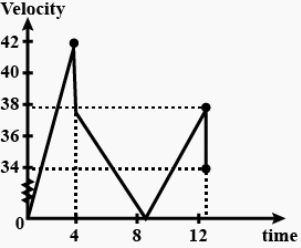Question13.Explain clearly, with examples, the distinction between:
a.  magnitude of displacement (sometimes called distance) over an interval of time, and the total length of path covered by a particle over the same interval;
b.  magnitude of average velocity over an interval of time, and the average speed over the same interval. [Average speed of a particle over an interval of time is defined as the total path length divided by the time interval]. Show in both (a) and (b) that the second quantity is either greater than or equal to the first.
When is the equality sign true? [For simplicity, consider one-dimensional motion only].

Solution :Let us consider an example of a football, it is passed to player B by player A and then instantly kicked back to player A along the same path. Now, the magnitude of displacement of the ball is 0 because it has returned to its initial position. However, the total length of the path covered by the ball = AB +BA = 2AB. Hence, it is clear that the first quantity is greater than the second.

( b ) Taking the above example, let us assume that football takes t seconds to cover the total distance. Then,

The magnitude of the average velocity of the ball over time interval t = Magnitude of displacement/time interval

= 0 / t = 0.

The average speed of the ball over the same interval = total length of the path/time interval

= 2AB/t

Thus, the second quantity is greater than the first.

The above quantities are equal if the ball moves only in one direction from one player to another (considering one-dimensional motion).

Question14.A man walks on a straight road from his home to a market 2.5 km away with a speed of 5 km h –1. Finding the market closed, he instantly turns and walks back home with a speed of 7.5 km h–1. What is the
a. magnitude of average velocity, and
b.  average speed of the man over the interval of time (i) 0 to 30 min, (ii) 0 to 50 min, (iii) 0 to 40 min? [Note: You will appreciate from this exercise why it is better to define average speed as total path length divided by time, and not as magnitude of average velocity. You would not like to tell the tired man on his return home that his average speed was zero!]

Solution :

Distance to the market = 2.5 km = 2500 m

Speed of the man  walking to the market= 5 km/h = 5 x (5/18) = 1.388 m/s

Speed of the man walking when he returns back home = 7.5 km/h = 7.5  x (5/18) = 2.08 m/s

(a) Magnitude of the average speed is zero since the displacement is zero

(b)

(i) Time taken to reach the market = Distance/Speed = 2500/1.388 = 1800 seconds = 30 minutes

So, the average speed over 0 to 30 minutes is 5 km/h or  1.388 m/s

(ii) Time taken to reach back home = Distance/Speed = 2500/2.08 = 1200 seconds = 20 minutes

So, the average speed is

Average Speed over a interval of 50 minutes= distance covered/time taken = (2500 + 2500)/3000 = 5000/3000 = 5/3 = 1.66 m/s

= 6 km/h

(ii) Average speed over an interval of 0 – 40 minutes = distance covered/ time taken = (2500+ 1250)/2400 = 1.5625 seconds = 5.6 km/h

Question 15. Why is there no distinction required between instantaneous speed and magnitude of velocity?

Solution :
Instantaneous velocity is the first derivative of distance with respect to time (dx / dt). However, dt is so small it is assumed that the moving particle does not change direction. As a result, the total distance and the magnitude of displacement become equal in this time interval. Thus, instantaneous speed and magnitude of velocity are equal.

Question 16.Look at the graphs (a) to (d) (Fig. 3.20) carefully and state, with reasons, which of these cannot possibly represent one-dimensional motion of a particle.
a.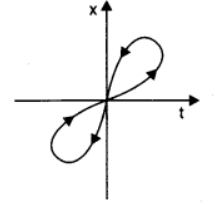b.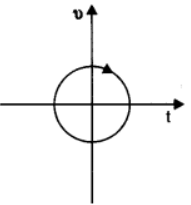c.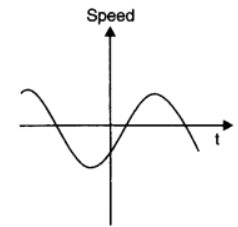d.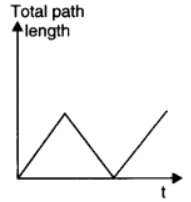Solution :
a. The given x-t graph, shown in (a), does not represent one-dimensional motion of the particle. This is because a particle cannot have two positions at the same instant of time.
b. The given v-t graph, shown in (b), does not represent one-dimensional motion of the particle. This is because a particle can never have two values of velocity at the same instant of time.
c.  The given v-t graph, shown in (c), does not represent one-dimensional motion of the particle. This is because speed being a scalar quantity cannot be negative.
d. The given v-t graph, shown in (d), does not represent one-dimensional motion of the particle. This is because the total path length travelled by the particle cannot decrease with time.

Question 17.Figure 3.21 shows the x-t plot of one-dimensional motion of a particle. Is it correct to say from the graph that the particle moves in a straight line for t < 0 and on a parabolic path for t > 0? If not, suggest a suitable physical context for this graph.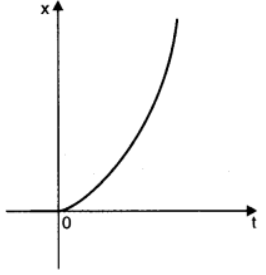(Fig 3.21)

Solution :
No
The x-t graph of a particle moving in a straight line for t < 0 and on a parabolic path for t > 0 cannot be shown as the given graph. This is because, the given particle does not follow the trajectory of path followed by the particle as t = 0, x = 0. A physical situation that resembles the above graph is of a freely falling body held for sometime at a height

Question18.A police van moving on a highway with a speed of 30 km h–1 fires a bullet at a thief’s car speeding away in the same direction with a speed of 192 km h–1. If the muzzle speed of the bullet is 150 m s–1, with what speed does the bullet hit the thief’s car ? (Note: Obtain that speed which is relevant for damaging the thief’s car).

Solution :
Speed of the police van, vp = 30 km/h = 8.33 m/s
Muzzle speed of the bullet, vb = 150 m/s
Speed of the thief’s car, vt = 192 km/h = 53.33 m/s
Since the bullet is fired from a moving van, its resultant speed can be obtained as:
= 150 + 8.33 = 158.33 m/s
Since both the vehicles are moving in the same direction, the velocity with which the bullet hits the thief’s car can be obtained as:
vbt = vb – vt
= 158.33 – 53.33 = 105 m/s

Question 19.Suggest a suitable physical situation for each of the following graphs (Fig 3.22):
a.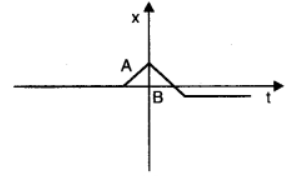b.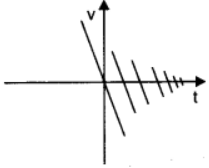c.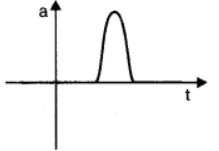(Fig: 3.22)

Solution :
a. The given x-t graph shows that initially a body was at rest. Then, its velocity increases with time and attains an instantaneous constant value. The velocity then reduces to zero with an increase in time. Then, its velocity increases with time in the opposite direction and acquires a constant value. A similar physical situation arises when a football (initially kept at rest) is kicked and gets rebound from a rigid wall so that its speed gets reduced. Then, it passes from the player who has kicked it and ultimately gets stopped after sometime.

b. In the given v-tgraph, the sign of velocity changes and its magnitude decreases with a passage of time. A similar situation arises when a ball is dropped on the hard floor from a height. It strikes the floor with some velocity and upon rebound, its velocity decreases by a factor. This continues till the velocity of the ball eventually becomes zero.

c. The given a-t graph reveals that initially the body is moving with a certain uniform velocity. Its acceleration increases for a short interval of time, which again drops to zero. This indicates that the body again starts moving with the same constant velocity. A similar physical situation arises when a hammer moving with a uniform velocity strikes a nail.

Question 20.Figure 3.23 gives the x-t plot of a particle executing one-dimensional simple harmonic motion. (You will learn about this motion in more detail in Chapter14). Give the signs of position, velocity and acceleration variables of the particle at t = 0.3 s, 1.2 s, – 1.2 s.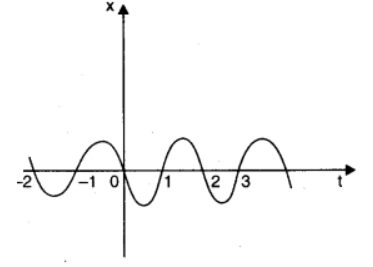(Fig: 3.23)

Solution :
Negative, Negative, Positive (at t = 0.3 s)
Positive, Positive, Negative (at t = 1.2 s)
Negative, Positive, Positive (at t = –1.2 s)
For simple harmonic motion (SHM) of a particle, acceleration (a) is given by the relation:
a = – ω2x ω → angular frequency … (i)
t = 0.3 s
In this time interval, x is negative. Thus, the slope of the x-t plot will also be negative. Therefore, both position and velocity are negative. However, using equation (i), acceleration of the particle will be positive.
t = 1.2 s
In this time interval, x is positive. Thus, the slope of the x-t plot will also be positive. Therefore, both position and velocity are positive. However, using equation (i), acceleration of the particle comes to be negative.
t = – 1.2 s
In this time interval, x is negative. Thus, the slope of the x-t plot will also be negative. Since both x and t are negative, the velocity comes to be positive. From equation (i), it can be inferred that the acceleration of the particle will be positive.

Question 21:Figure 3.24 gives the x-t plot of a particle in one-dimensional motion. Three different equal intervals of time are shown. In which interval is the average speed greatest, and in which is it the least? Give the sign of average velocity for each interval.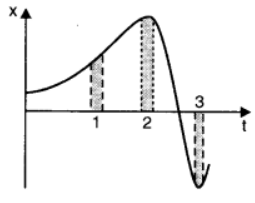(Fig: 3.24)

Solution :
Interval 3 (Greatest), Interval 2 (Least)
Positive (Intervals 1 & 2), Negative (Interval 3)
The average speed of a particle shown in the x-t graph is obtained from the slope of the graph in a particular interval of time.
It is clear from the graph that the slope is maximum and minimum restively in intervals 3 and 2 respectively. Therefore, the average speed of the particle is the greatest in interval 3 and is the least in interval 2. The sign of average velocity is positive in both intervals 1 and 2 as the slope is positive in these intervals. However, it is negative in interval 3 because the slope is negative in this interval.

Question 22.Figure 3.25 gives a speed-time graph of a particle in motion along a constant direction. Three equal intervals of time are shown. In which interval is the average acceleration greatest in magnitude? In which interval is the average speed greatest? Choosing the positive direction as the constant direction of motion, give the signs of v and a in the three intervals. What are the accelerations at the points A, B, C and D?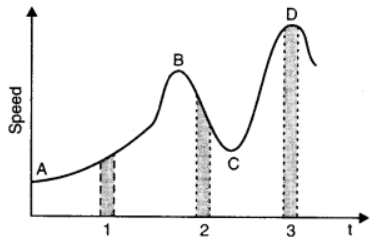(Fig: 3.25)

Solution :
Average acceleration is greatest in interval 2
Average speed is greatest in interval 3
v is positive in intervals 1, 2, and 3
a is positive in intervals 1 and 3 and negative in interval 2
a = 0 at A, B, C, D
Acceleration is given by the slope of the speed-time graph. In the given case, it is given by the slope of the speed-time graph within the given interval of time.
Since the slope of the given speed-time graph is maximum in interval 2, average acceleration will be the greatest in this interval.
Height of the curve from the time-axis gives the average speed of the particle. It is clear that the height is the greatest in interval 3. Hence, average speed of the particle is the greatest in interval 3.

In interval 1:
The slope of the speed-time graph is positive. Hence, acceleration is positive. Similarly, the speed of the particle is positive in this interval.

In interval 2:
The slope of the speed-time graph is negative. Hence, acceleration is negative in this interval. However, speed is positive because it is a scalar quantity.

In interval 3:
The slope of the speed-time graph is zero. Hence, acceleration is zero in this interval. However, here the particle acquires some uniform speed. It is positive in this interval.
Points A, B, C, and D are all parallel to the time-axis. Hence, the slope is zero at these points. Therefore, at points A, B, C, and D, acceleration of the particle is zero.

Question 23.A three-wheeler starts from rest, accelerates uniformly with 1 m s–2 on a straight road for 10 s, and then moves with uniform velocity. Plot the distance covered by the vehicle during the nth second (n = 1,2,3….) versus n. What do you expect this plot to be during accelerated motion: a straight line or a parabola?

Solution :

For a straight line, the distance covered by a body in nthsecond is :
SN = u + a (2n – 1)/2     . . . . . . . . ( 1 )
Where,

a = Acceleration

u = Initial velocity
n = Time = 1, 2, 3, . . . . . , n
In the above  case,
a = 1 m/s2 and u = 0.
∴ SN = (2n – 1) / 2     . . . . . . . . . . .( 2 )
This relation shows that:
SN ∝ n     . . . . .. . . . . ( 3 )

Now substituting different values of n in equation ( 2 ) we get:

 n 1 2 3 4 5 6 7 8 9 SN 0.5 1.5 2.5 3.5 4.5 5.5 6.5 7.5 8.5

The plot between n and Dn will be a straight line as shown: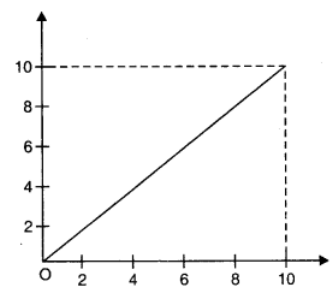This plot is expected to be a straight line.

Question24. A lady stands in an elevator which is open from above. She then throws a ball up with an initial speed of 40 m/s. After how long will the ball return to her hand?  The elevator then starts moving upwards with a uniform speed of 5 m s-1 , the lady again throws the ball up with the same initial speed, after how long will the ball return to her hand?

Solution :
Case 1 when the elevator is still:
We know,
v = u + at
0 = 40 + (- 9.8 )t   (Since final velocity = 0 and a = -g as gravity acts downwards)
-40/-9.8 = t
t = 4.081 s.
Thus, the total time taken by the ball to go up and return back is 4.081 x 2 = 8.16 s
Case 2 when the elevator is moving upwards :
The elevator moves up at a constant speed thus the relative velocity of the ball with respect to the lady remains the same. Thus it takes 8.16 s  for the ball to go up and down.

QuestionQuestion 25. On a moving walk way ( belt speed = 5kmph ) a child runs to and fro at a speed of 10 km h-1 (with respect to the belt) between his mother and father located 40 m apart on the moving belt. For an observer sitting in the lobby outside, what is the
a.  speed of the child running against the belt?
b.  speed of the child running in the same direction as the walk way?.
c.  time taken by the child in ( a ) and ( b )?
d.  Which of the answers change if the observer is either of the parents?

Solution :
Given,
Speed of the child with respect to the walk way = 10 kmph
Speed of the belt ( walk way ) = 5 kmph
a. When the child runs against the belt, then his speed with respect to the stationary observer = 10 – 5 = 5 kmph
b. When the child runs on the belt in the same direction as the belt, then his speed to the stationary observer  = 10+ 5 = 15 kmph
c. Distance between the parents = 40 m
As both the parents are on the walk way the speed of child remains the same for both the parents = 10 kmph = 2.77 m/s
Hence the time taken by the child to move to any one of his parent from another one = 40 / 2.77 = 14.44 s
d. For any of the parent as the observer, the answer to ( a ) and ( b ) changes while the answer to ( c ) is the same.

Question26. Hagrid throws two stones simultaneously from a treetop that is 200m above the jungle floor. The stones have an initial velocity of 15 m/s and 30 m/s. Does the graph, given below, correctly represent the time variation of the relative position of the second stone with respect to the first? Taking ‘g’ as 10m/s2, consider that there is no air resistance and that the stones come to a complete stop on hitting the jungle floor. Also, give the linear equations for the curved and linear parts of the graph.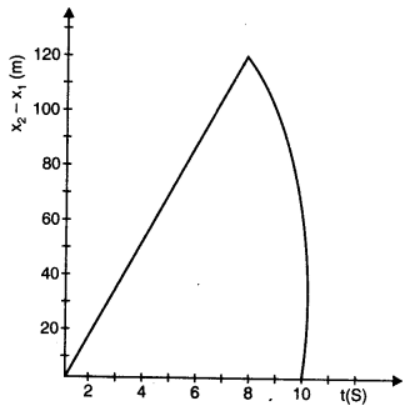Solution :
For the first stone:
Given,
Acceleration, a = –g = – 10 m/s2
Initial velocity, uI = 15 m/s
Now, we know
s1 = s0 + u1t + (1/2)at2
Given, height of the tree, s0 = 200 m
s1 = 200 + 15t – 5t2      . . . . . . . . .  . ( 1 )
When this stone hits the jungle floor, s1 = 0
∴– 5t2 + 15t + 200 = 0
t2 – 3t – 40 = 0
t2 – 8t + 5t – 40 = 0
t (t – 8) + 5 (t – 8) = 0
t = 8 s or t = – 5 s
Since, the stone was thown at time t = 0, the negative sign is not possible
∴t = 8 s
For second stone:
Given,
Acceleration, a = – g = – 10 m/s2
Initial velocity, uII = 30 m/s
We know,
s2 = s0 + uIIt + (1/2)at2
= 200 + 30t – 5t2 . . . . . . . . .  . . .  . ( 2 )
when this stone hits the jungle floor; s2 = 0
– 5t2 + 30 t + 200 = 0
t2 – 6t – 40 = 0
t2 – 10t + 4t + 40 = 0
t (t – 10) + 4 (t – 10) = 0
t (t – 10) (t + 4) = 0
t = 10 s or t = – 4 s
Here again, the negative sign is not possible
∴ t = 10 s
Subtracting equations ( 1 ) from equation ( 2 ), we get
s2 – s1 = (200 + 30t -5t2) – (200 + 15t -5t2)
s2 – s1 =15t   . . .  . . . . . . . . . .. . . . . . ( 3 )
Equation ( 3 ) represents the linear trajectory of the two stone, because to this linear relation between (s2 – s1) and t,,  the projection is a straight line till 8 s.
The maximum distance between the two stones is at t = 8 s.
(s2 – s1)max = 15× 8 = 120 m
This value has been depicted correctly in the above graph.
After 8 s, only the second stone is in motion whose variation with time is given by the quadratic equation:
s2 – s1 = 200 + 30t – 5t2
Therefore, the equation of linear and curved path is given by :
s2 – s1 = 15t (Linear path)
s2 ­– s1 = 200 + 30t – 5t2 (Curved path)

Question27.The given speed- time graph represents the motion of a particle in a fixed direction. Calculate the distance covered by the particle in the time intervals; ( a ) t = 0 s to 10 s, (b) t = 1 s to 8 s. Find the average speed of the particle over the intervals in ( a ) and ( b ).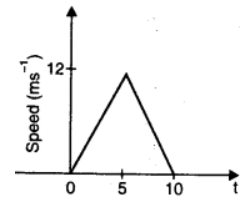Solution :
( a ) Distance covered by the particle = Area of the given graph
= (1/2)base x height
= (1/2) x (10) x (12) = 60m
Average speed of the particle = 60/10 = 6 m/s
( b )The distance traversed by the particle between
t = 1s to 8s
let distance travelled in 1 to 5s be S1 and distance travelled from 6 to 8s be S2.
Thus, total distance travelled, S ( in t = 1 to 8 s) = S1 + S2  . . . . . . ( 1 )
Now, For S1.
Let u’ be the velocity of the particle after 1 second  and a’ be the acceleration in the particle from t = 0 to 5s
We know that the particle is under uniform acceleration from  t = 0 to 5s thus, we can obtain  acceleration using the first equation of motion.
v = u + at
where, v = final velocity
12 = 0 + a’(5)
a’ = 2.4 m/s2
Now to find the velocity of the particle at 1s
v = 0 + 2.4 (1)
v =  2.4 m/s = u’ at t = 1s
Thus, the distance covered by the particle in 4 seconds  i.e., from t = 1 to 5 s.
S1 = u’t  +  ½ a’t2
=  2.4 x 4  +  ½ x 2.4 x 42
= 9.6 + 19.2 =28.8 m
Now, for S2
Let a’’ be the uniform acceleration in the particle from 5s to 10s
Using the first law of motion
v = u + at  . . . . . . . ( v= 0 as the particle comes to rest )
0 = 12 + a’’ x 5
a’’ = -2.4 m/s
Thus, distance travelled by the particle in 3 seconds i.e., between 5s to 8s
S2 = u’’t + ½ a’’t
S2 = 12 x 3 + ½ x(-2.4) x 32
=  36 + (-1.2)x9
S2 = 25.2m
Thus, putting the values of S1 and S2 in equation ( 1 ), we get:
S = 28.8 + 25.2 = 54m
Therefore, average speed = 54 / 7 = 7.71 m/s.

Question28. The graph below is a velocity-time graph of a particle in one-dimensional motion. Which of the following formulae correctly describe the motion of the particle in the time interval t1 to t2.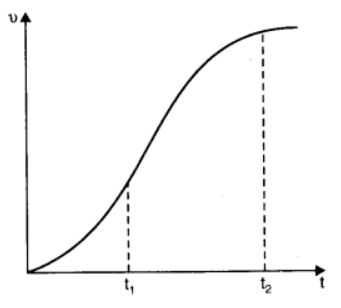a. aaverage = (v(t2) – v(t1))/(t2 – t1)
b. x(t2 ) = x(t1) + vaverage (t2 – t1) + (½) aaverage (t2 – t1)2
c.  vaverage = (x(t2) – x(t1))/(t2 – t1)
d. v(t2 ) = v(t1) + a (t2 – t1)
e. x(t2 ) = x(t1) + v (t1) (t2 – t1) +(½) a (t2 – t1)2
f.  x(t2 ) – x(t1) = area under the v-t curve bounded the dotted line and by the t-axis.

Solution :
The correct formulae describing the motion of the particle are (c), (d) and, (f)
The given graph has a non-uniform slope. Hence, the formulae given in (a), (b), and (e) cannot describe the motion of the particle. Only relations given in (c), (d), and (f) are correct equations of motion.